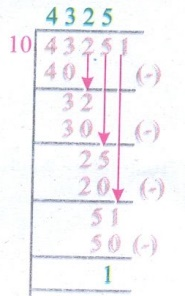Home | | Maths 5th Std | Exercise 2.8 (Division)

# Exercise 2.8 (Division)

Text Book Back Exercises Questions with Answers, Solution : 5th Maths : Term 1 Unit 2 : Numbers : Exercise 2.8 (Division)

Exercise 2.8

1. Find quotient and remainder

a. 5732 ÷ 9

b. 47345 ÷ 5

c. 3032 ÷ 7

d. 43251 ÷ 10

e. 2532 ÷ 4

a. 5732 ÷ 9Quotient = 636

Remainder = 8

b. 47345 ÷ 5Quotient = 9469

Remainder = 0

c. 3032 ÷ 7Quotient = 433

Remainder = 1

d. 43251 ÷ 10Quotient = 4325

Remainder = 1

e. 2532 ÷ 4Quotient = 633

Remainder = 0

a) 3057 families are living in a town. The town panchatyat decided to split the town into 3 wards. How many families will be there in each panchayat?

Families in the town = 3057

Families in 3 wards = 3057

Families in 1 ward = 3057 ÷ 3Families in 1 ward = 1019

b) A water board shares 28,049 litres daily to 7 lorries. How much of water is given to each lorry?

Water given to 7 lorries = 28,049 litres

Water given to each lorry = 28,049 ÷ 7Water given to each lorry = 4007L

c) A company gives ₹ 93,300 as salary for 6 workers. Find the salary of one worker.

Salary given for 6 workers = ₹ 93,300

Salary of one worker = ₹ 93,300 ÷ 6Salary for one worker = 15,550

Tags : Numbers | Term 1 Chapter 2 | 5th Maths , 5th Maths : Term 1 Unit 2 : Numbers
Study Material, Lecturing Notes, Assignment, Reference, Wiki description explanation, brief detail
5th Maths : Term 1 Unit 2 : Numbers : Exercise 2.8 (Division) | Numbers | Term 1 Chapter 2 | 5th Maths